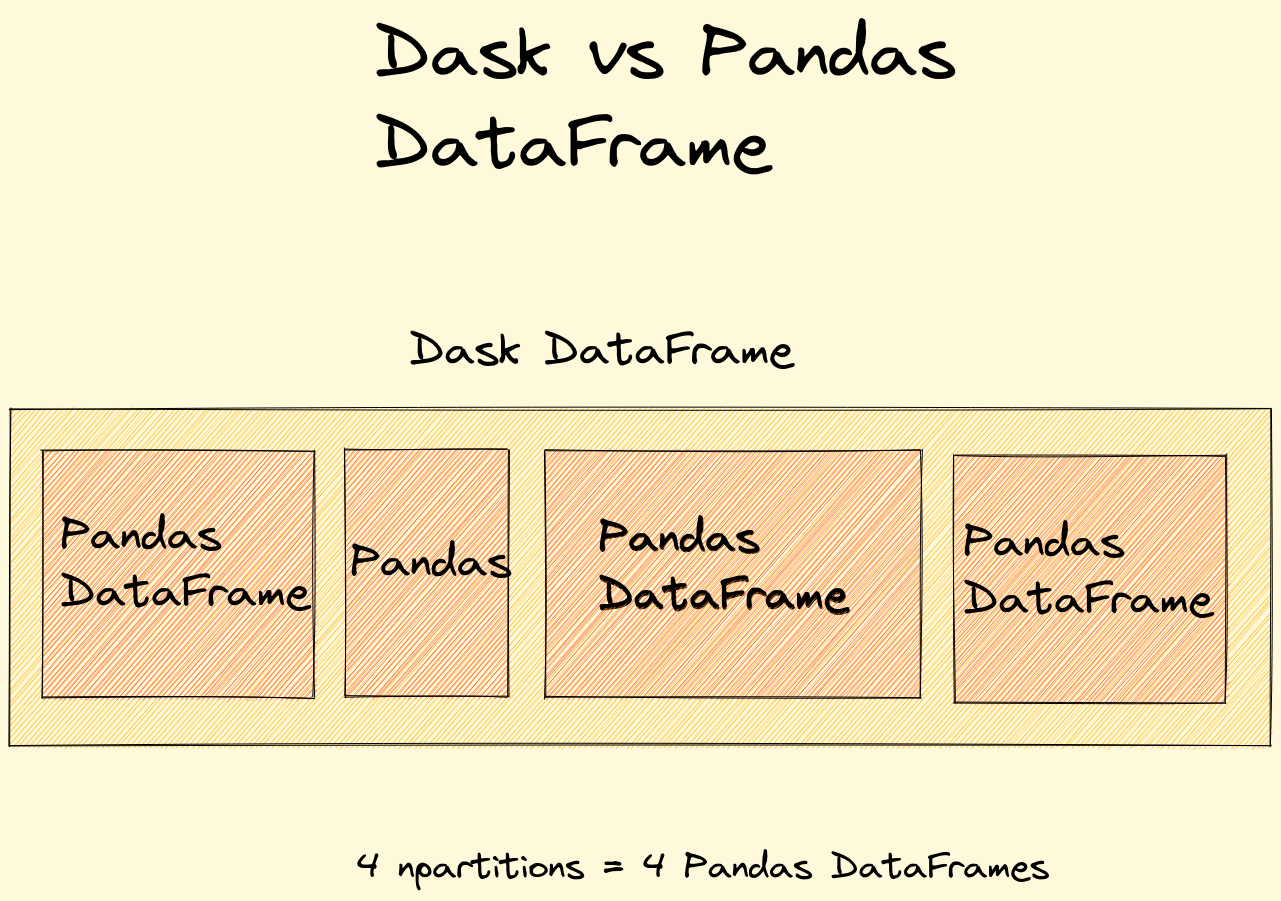In this short article, we will see how to convert Pandas DataFrame to Dask DataFrame. We will also cover conversion from Dask to Pandas DataFrame.

(1) Convert Pandas to Dask DataFrame

``````from dask import dataframe as dd
df_dd = dd.from_pandas(df, npartitions=2)
``````

(2) Convert Dask to Pandas DataFrame

``````df_dd.compute()
``````

Let's cover both cases in examples and more details.

There is nice reading from Tom Augspurger Modern Pandas (Part 8): Scaling.

You can't have a DataFrame larger than your machine's RAM. In practice, your available RAM should be several times the size of your dataset

So one of the problems with Pandas is the large datasets. On the other hand, Dask allows you to have a DataFrame larger than your RAM.

And another important information:

A Dask DataFrame consists of many pandas DataFrames arranged by the index. Dask is really just coordinating these pandas DataFrames.

So Dask DataFrame consists of Pandas DataFrames arranged by the index.Use Pandas when your machine has enough memory. Otherwise use Dask DataFrame and if needed extract a smaller subset from the Dask DataFrame.

Both share very common API - if you like to find how to work with Dask please read: How to Read and Analyze a Large CSV File With Pandas/Dask

## 1: Convert Pandas to Dask DataFrame

First we will see how to Convert Pandas to Dask DataFrame. Suppose we have Pandas DataFrame as the one below:

``````import pandas as pd

data = {'A':[11,12,13],'B':[21,22,23],'C':[31,32,33]}
df = pd.DataFrame(data)
``````

Which looks like:

A B C
0 11 21 31
1 12 22 32
2 13 23 33

### 1.1 Convert Pandas to Dask

To convert it to Dask DataFrame we can use Dask method `.from_pandas()`:

``````from dask import dataframe as dd
df_dd = dd.from_pandas(df, npartitions=3)
``````

This give us:

``````Dask DataFrame Structure:
A      B      C
npartitions=2
0              int64  int64  int64
1                ...    ...    ...
2                ...    ...    ...
``````

We can see the types of both DataFrames by:

``````type(df_dd)
type(df)
``````

and result is:

``````dask.dataframe.core.DataFrame
pandas.core.frame.DataFrame
``````

### 1.2 dd.from_pandas() parameters

The method has signature:

``````dd.from_pandas(
data,
npartitions=None,
chunksize=None,
sort=True,
name=None,
)
``````

where important are 2 parameters:

• `npartitions` - number of partitions
• `chunksize` - size of each partition

### 1.3 ValueError: Exactly one of npartitions and chunksize must be specified

For example error like:

ValueError: Exactly one of npartitions and chunksize must be specified.

is raised when on of:

• `npartitions`
• `chunksize`

is missing.

So at this point when you convert you need to know:

• how many partitions (sub Pandas DataFrames ) there will be
• what is the size for those sub Pandas DataFrames which makes the Dask DataFrame

• system memory
• original dataset/DataFrame memory
• what operations will be done on the Dask DataFrame

## 2: Convert Dask to Pandas DataFrame

To convert from Dask to Pandas DataFrame we can use method `.compute()`:

``````df_pd = df_dd.compute()
``````

Now we get again:

A B C
0 11 21 31
1 12 22 32
2 13 23 33

And the types are:

``````type(df_dd)
type(df_pd)
``````

and result is:

``````dask.dataframe.core.DataFrame
pandas.core.frame.DataFrame
``````